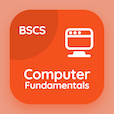SAT Online Courses

SAT Chemistry Quizzes

SAT Chemistry Quiz PDF - Complete

# Empirical Formula Multiple Choice Questions p. 11

Study Empirical Formula multiple choice questions and answers, empirical formula quiz answers PDF 11 to study SAT Chemistry course online. Mole Concept MCQ trivia questions, Empirical Formula Multiple Choice Questions (MCQ) for online college degrees. "Empirical Formula MCQ" PDF eBook: quantum numbers and energy levels, safety, compounds of environmental concern, introduction, empirical formula test prep for online SAT prep.

"In combustion analysis, % of hydrogen is calculated from" MCQ PDF: co, ch, h2o, and co2 to apply to colleges online. Learn mole concept questions and answers to improve problem solving skills for college entrance examination.

## Empirical Formula Questions and Answers MCQs

MCQ: In combustion analysis, % of hydrogen is calculated from

CH
CO
H2O
CO2

MCQ: The combustion of carbon in oxygen is an example of

Endothermic reaction
Exothermic reaction
Displacement reaction
Neutralization reaction

MCQ: Chromium VI is highly toxic causing disease of

Kidney
Lungs
Nervous system
Cancer

MCQ: In case of very small burns, the burnt place should be dipped in

8 % NaHCO3 solution
9 % NaHCO3 solution
10 % NaHCO3 solution
20 % NaHCO3 solution

MCQ: The quantum number that gives orientation of orbitals in space is

Principal quantum number
Spin quantum number
Magnetic quantum number
Azimuthal quantum number

### More Quizzes from SAT Chemistry Course